# 2980. 小数转化分数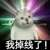from：https://wenku.baidu.com/view/44e00a25ddccda38366baf0d.html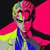### include

typedef long long ll;
using namespace std;
string s;
void solve()
{
if(s.find("[")==string::npos){
ll n=s.find(".");
ll len=s.size()-n-1;
s=s.erase(s.find("."),1);
ll f=0;ll g=1;
for(ll i=0;i<s.size();i++) f=f*10+(s[i]-'0');
for(ll i=1;i<=len;i++) g*=10;
ll k=gcd(f,g);
cout<<f/k<<"/"<<g/k<<endl;
}
else{
ll pos1=s.find("["),pos2=s.find("]");
ll n=s.find(".");
if(n==pos1-1){
ll f=0,g=0,zheng=0;
string str=s.substr(pos1+1,pos2-pos1-1);
for(ll i=0;i<str.size();i++){
f=f*10+(str[i]-'0');
}
for(ll i=0;i<str.size();i++){
g=g*10+9;
}
ll k=gcd(f,g);
f=f/k,g=g/k;
for(ll i=0;i<n;i++){
zheng=zheng*10+s[i]-'0';
}
f=f+zheng*g;
k=gcd(f,g);
cout<<f/k<<"/"<<g/k<<endl;
}
else{
string str=s.substr(pos1+1,pos2-pos1-1);
ll f=0,g=0,zheng=0;
string ssd=s.substr(n+1,pos1-n-1);
string spw=ssd+str;
string spp=s.substr(0,n);
for(ll i=0;i<spp.size();i++) zheng=zheng*10+spp[i]-'0';
ll q=0,p=0;
for(ll i=0;i<spw.size();i++) q=q*10+spw[i]-'0';
for(ll i=0;i<ssd.size();i++) p=p*10+ssd[i]-'0';
f=q-p;
for(ll i=0;i<str.size();i++) g=g*10+9;
for(ll i=0;i<ssd.size();i++) g*=10;
ll k=gcd(f,g);
f=f/k,g=g/k;
f=f+g*zheng;
k=gcd(f,g);
cout<<f/k<<"/"<<g/k<<endl;
}
}
}
int main()
{
int t;cin>>t;
for(int j=0;j<t;j++){
cin>>s;
cout<<"case #"<<j<<":\n";
solve();
}
}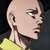#include <bits/stdc++.h>

using namespace std;

typedef long long LL;

LL gcd(LL a, LL b)
{
if(a > b)
{
LL c = a % b;
while(c)
{
a = b;
b = c;
c = a % b;
}
return b;
}
else
return gcd(b, a);
}

void solve()
{
string s;
cin >> s;
int jdg = 0;
for(int i = 0; i < s.size(); i++)
{
if(s[i] == '[')
{
jdg = 1;
break;
}
}
LL up = 0, down = 0 , up1 = 0, down1 = 0;//up1与down1为循环小数准备

int pos;//记录小数点的位置
int pos1, pos2;//记录【】分别的位置

if(jdg == 0)//有限小数
{
for(int i = 0; i < s.size(); i++)
{
if(s[i] == '.')
{
pos = i;
break;
}
}
for(int i = 0; i < s.size(); i++)
{
if(i == pos)
continue;
else
up = up * 10 + s[i] - '0';
}
down = pow (10, s.size() - 1 - pos);
cout << up / gcd(up, down) << '/' << down / gcd(up, down) << endl;
}
else//循环小数
{
for(int i = 0; i < s.size(); i++)
{
if(s[i] == '.')
pos = i;
if(s[i] == '[')
pos1 = i;
if(s[i]==']')
pos2 = i;
}
for(int i = 0; i < pos1; i++)
{
if(i == pos)
continue;
else
up = up * 10 + s[i] - '0';
}
for(int i = pos1 + 1; i < pos2; i++)
{
up1 = up1 * 10 + s[i] - '0';
}
down1 = pow (10, pos2 - pos1 - 1) - 1;
if(pos == pos1 - 1)
{
up1 = up1 + up * down1;
cout << up1 / gcd(up1, down1)<< '/' << down1 / gcd(up1, down1) << endl;
}
else
{
up1 = up1 + up * down1;
down1 = down1 * pow(10, pos1 - pos - 1);
cout << up1 / gcd(up1, down1) << '/' << down1 / gcd(up1, down1) << endl;
}
}
}
int main()
{
int t;
cin >> t;
for(int i = 0; i < t; i++)
{
cout << "case #" << i << ":\n";
solve();
}
return 0;
}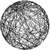stoull是个好东西，可以省去不少手动string转int的麻烦

#include<bits/stdc++.h>
using namespace std;
using u64 = uint64_t;
int main()
{
int T; cin >> T;
for(int cs = 0; cs < T; cs++)
{
cout << "case #" << cs << ":\n";
string s; cin >> s;
auto dot = s.find('.');
u64 a = stoull(s.substr(0, dot)), n, m;
auto rec = s.find('[');
if(rec == s.npos)
{ // 有限小数
n = stoull(s.substr(dot + 1));
m = u64(pow(10, s.length() - dot - 1));
}
else
{ // 循环小数
s.erase(rec, 1);
n = stoull(s.substr(dot + 1));
if(dot + 1 != rec)n -= stoull(s.substr(dot + 1, rec - dot - 1));
m = u64(pow(10, s.length() - rec - 1)) - 1;
m *= u64(pow(10, rec - dot - 1));
}
auto g = gcd(n, m); n /= g; m /= g;
cout << n + m * a << "/" << m << "\n";
}

}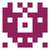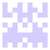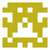#include <cstddef>
#include <iostream>
#include <istream>
#include <cmath>
#include <string>
using namespace std;

struct rational{
string origindata;
string loop; //循环节
string inter;//整数部分
string decimal;//小数部分
long int numer;
long int denom;
bool isLoop;
friend istream& operator>>(istream& is,rational& rat);
friend ostream& operator<<(ostream& os,const rational& rat);
void convert();
};
long gcd(long a,long b);
int main(){
rational rat;
int T;
cin >> T;
for(int i = 0;i < T;i++){
cin >> rat;
cout << "case #"<<i<<":\n"<<rat<<endl;
}
return 0;
}

istream& operator>>(istream& is,rational& rat){
is >> rat.origindata;
size_t dotpos = rat.origindata.find('.');
size_t looppos = rat.origindata.find('[');
//整数部分
if(dotpos == string::npos){
rat.inter = rat.origindata;
}else{
rat.inter = rat.origindata.substr(0,dotpos);
}

//小数部分
if(looppos == string::npos){
rat.isLoop = false;
if(dotpos != string::npos)
rat.decimal = rat.origindata.substr(dotpos+1);
}else{
rat.isLoop = true;
size_t endloop = rat.origindata.find(']');
rat.decimal = rat.origindata.substr(dotpos+1,looppos - dotpos - 1);
rat.loop = rat.origindata.substr(looppos+1,endloop - looppos - 1);
}
rat.convert();
return is;
}
ostream& operator<<(ostream& os,const rational& rat){
if(rat.denom != 1)
os << rat.numer <<'/'<< rat.denom;
else
os << rat.numer;
return os;
}
void rational::convert(){
// cout << inter <<' ' << decimal << ' ' << loop << endl;
long a = pow(10,decimal.length());
long  b = pow(10,loop.length());
long small = atol(inter.data())*a + atol(decimal.data());
long big   = isLoop?small*b+atol(loop.data()):small * 10;
numer = big - small;
denom = isLoop?b*a - a:a*9;
long g = gcd(numer,denom);
numer /= g;
denom /= g;
}

long gcd(long a,long b){
return b ? gcd(b,a%b):a;
}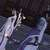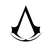#include<bits/stdc++.h>
using namespace std;
long long int gcd(long long int a,long long int b)
{
if(a%b==0) return b;
else return gcd(b,a%b);
}
void solve()
{
char s;
scanf("%s",s);
int l=strlen(s);
double num=0;
int d=1;
int flag=1;
int qi=0;
int jie=l;
int chu=0;
for(int i=0;i<l;i++)
{
if(isdigit(s[i])==1&&flag==1) num=num*10+s[i]-'0';
else if(isdigit(s[i])==1&&flag==0) num=num+(s[i]-'0')/pow(10,d),d++;
else if(s[i]=='.') flag=0,qi=i+1;
else if(s[i]=='[') qi=i+1,chu=1;
else if(s[i]==']') jie=i;
//cout<<i<<ends<<d<<ends<<num<<endl;
}
int k=jie-qi;
double num2=num*pow(10,k);
//cout<<d<<endl;
if(chu==0)
{
double k1=pow(10,k);

long long int num3=num2;
long long int k2=k1;
long long int g=gcd(num3,k2);
//cout<<num3<<ends<<k2<<endl;
cout<<num3/g<<"/"<<k2/g<<endl;
}
else
{
double k1=pow(10,k)-1;

int wei=d-k;
for(int j=qi;j<jie;j++)
{
num2+=(s[j]-'0')/pow(10,wei++);

}
num2-=num;
num2*=pow(10,d-k);
k1*=pow(10,d-k);
// cout<<d<<ends<<k<<endl;
long long int num3=num2;
long long int k2=k1;
long long int g=gcd(num3,k2);
cout<<num3/g<<"/"<<k2/g<<endl;

}

}
int main()
{
int n;
cin>>n;
for(int i=0;i<n;i++)
{
printf("case #%d:\n",i);
solve();
}
}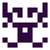6666666666666666666666666666666666666666666666666666666666666666666666666666666666666666666666666666666666666666[em:03]